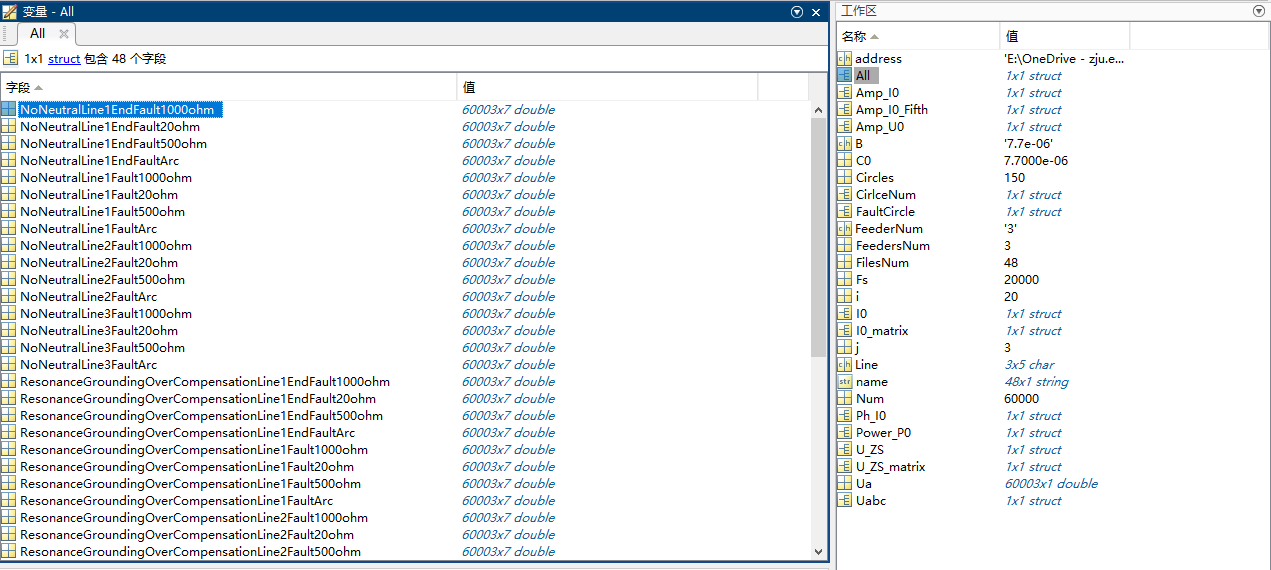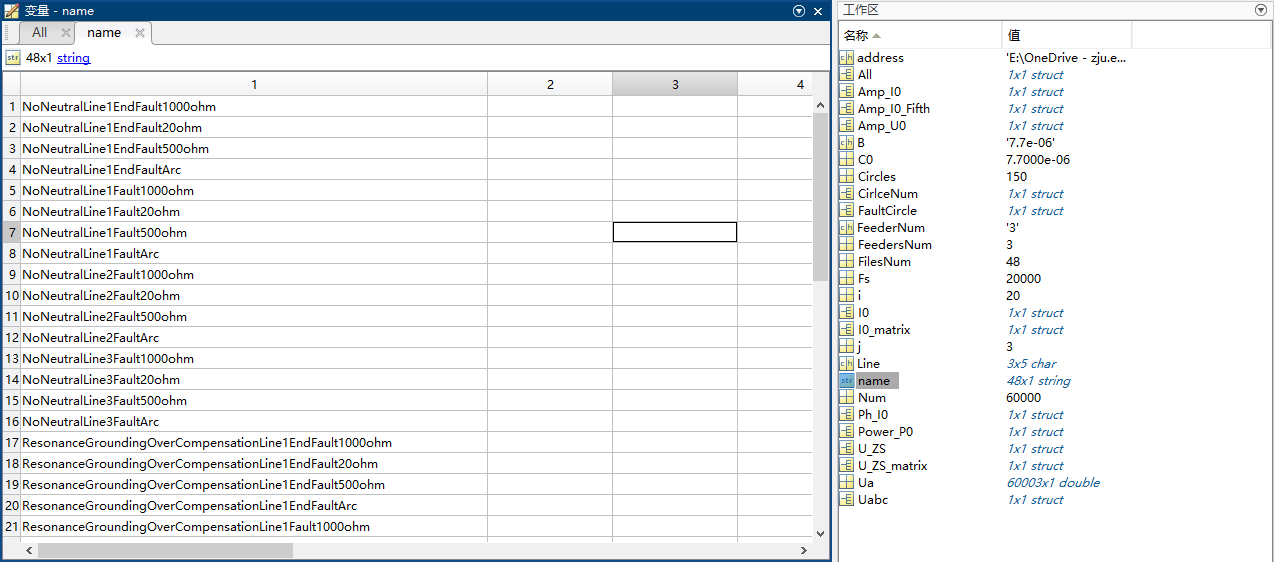Python学习纪要——把txt文件批量转换成csv

function [All,len,lable] = ReadAllCsv(address)

len = length(files);  % 获取当前文件的长度
%    M = 60; %设定数据文件名称最大值，对字符串进行补全，因为将多个不同长度的一维数组存入二维数组时，需要将所有一维数组的长度转为相同长度，可以采用在数组后边补零的方式实现。
for i = 1: len     % 开始循环
lable(i,:) = string(files(i).name(1:end-4));
str = strcat ('E:\OneDrive - zju.edu.cn\Simulation Models\2. PSCAD46\5. 为Tensorflow出仿真数据\仿真数据\',files(i).name);
%       str_var(i,:) = strcat('All_',lable(i,:));
%         name = strip(lable(i,:));
All.(lable(i,:)) = var;
end
end


lable(i,:) = files(i).name(1:end-4);


M = 60; %设定数据文件名称最大值，对字符串进行补全，因为将多个不同长度的一维数组存入二维数组时，需要将所有一维数组的长度转为相同长度，可以采用在数组后边补零的方式实现。